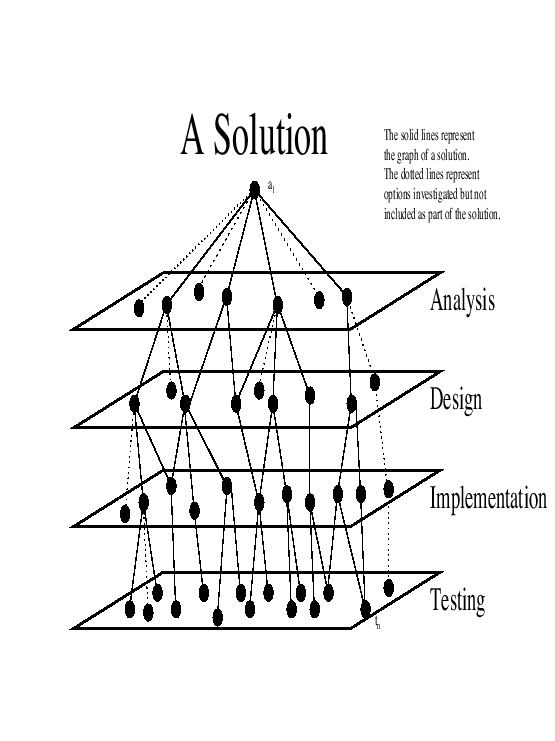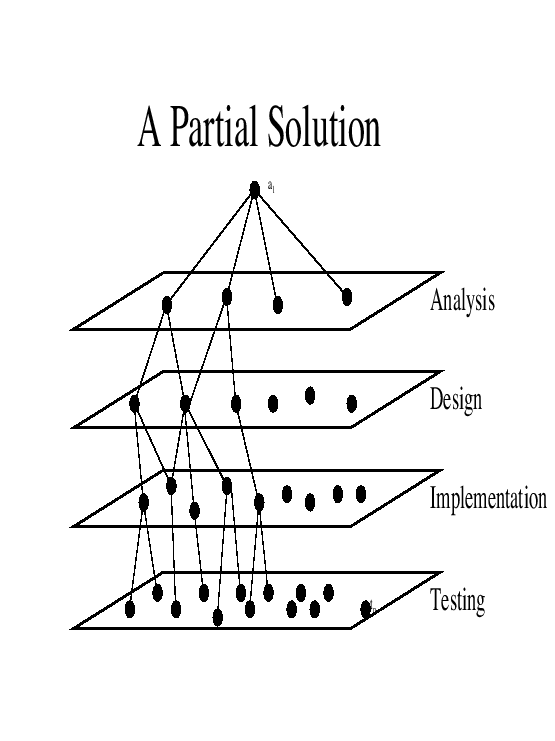Next: Towards the Definition of Up: Definitions Previous: Towards the Definition of

## Towards the Definition of Methodology

Next we define the methodology, as soon discussed, as an algorithm that finds a solution in the given environment of the multi-layered finite space consisting of the analysis, design, implementation, and testing plane, starting with the root represented by the problem statement and ending with the goal represented by the system acceptance test. Three classes of methodologies, or algorithms, are presented: sequential, cyclical, and the WaterSluice.

Definition 18 (Solution)   A solution is a tree, or in a more general case, a directed acyclic graph, rooted at the problem statement and includes the system acceptance test that satisfies all of the goals in the problem statement.

In a static environment, the solution may include all steps known in the environment. In a dynamic environment, the solution may be a subset. A solution does not contain any conflicting steps. The solution represents the final system, complete with analysis, design, implementation, and testing. A step visited but not used in the solution represents wasted effort. See Figure 5.7 on pagefor a visual representation of a solution.Definition 19 (Partial Solution)   A partial solution satisfies a consistent collection of goals in the problem statement.

A partial solution does not satisfy all of the goals in the problem statement. If some of the goals in the problem statement are in conflict with each other, then only a partial solution exists. See Figure 5.8 on pagefor a visual representation of a partial solution.Definition 20 (Optimal Solution)   An optimal solution satisfies all of the goals in the problem statement in an optimal fashion.

If some of the goals in the problem statement are in conflict with each other, then no optimal solution exists.

Definition 21 (Feasible Solution)   A feasible solution satisfies all of the goals in the problem statement but not necessarily in an optimal fashion.

There may be many feasible solutions to a given problem statement.

Assumption 1   The space is finite and of size N.

This thesis deals with software engineering of systems that can be built in a finite amount of time with finite resources using finite computers. Thus the assumption of a finite space is reasonable. Consider the alternative that the space is infinite. Software engineering systems simply can't be built that require an infinite number of steps.

This introduces the question of the size of this space. What is N?

Let N1 be the number of steps in an optimal solution. Recall that an optimal solution satisfies all the goals in the problem statement.

A feasible solution satisfies all of the goals in the problem statement but not necessarily in an optimal fashion. A feasible solution has the same starting problem statement and the same ending system acceptance test as an optimal solution. Let N2 be the upper bound on the number of steps over all feasible solution.

A partial solution satisfies some of the goals in the problem statement. A partial solution has the same ending system acceptance test as an optimal solution but satisfies only some of the goals of the problem statement. Let N3 be the upper bound on the number of steps over all permutations and sub collections of the goals from the problem statement over all partial solutions.

Define the size of the space to be the upper bound of N1, N2, and N3.

Definition 22 (Methodology)   A methodology is an algorithm that finds a feasible solution in the given environment of the multi-layered space consisting of the analysis, design, implementation, and testing plane, starting with the root represented by the problem statement and ending with the goal represented by the system acceptance test.

The three main categories of software engineering methodologies under investigation are sequential, cyclical, and the WaterSluice.

Definition 23 (Sequential Methodology)   In a sequential software engineering methodology, all steps in the analysis plane are completed first, followed by all steps in the design plane, followed by all steps in the implementation plane, and then followed by all steps in the testing plane.

A more detailed description can be found in Section 3.1. Also see Figure 3.1 on pagefor a graphical representation of a sequential methodology.

Definition 24 (Cyclical Methodology)   A cyclical software engineering methodology cycles through each phase a few steps at a time until a feasible solution is established.

Simply, a cyclical software engineering methodology iterates over the processes of think a little, plan a little, implement a little, then test a little. Finer and finer details are generated as the cyclical software engineering methodology progresses. Finally, after several iterations, the system is completed.

A more detailed description can be found in Section 3.2. Also see Figure 3.2 on pagefor a graphical representation of a cyclical methodology.

Definition 25 (WaterSluice)   The WaterSluice combines the steady progression of the sequential software engineering methodology with the iterative nature of the cyclical software engineering methodology while adding priority. Non-monotonic conflicts are handled by change order control.

The WaterSluice software engineering methodology separates the important aspects from the less important and concentrates on solving them first. As the process continues, finer and finer details are refined until the product is released.

A more detailed description can be found in Section 3.3.1. Also see Figure 3.4 on pagefor a graphical representation of the WaterSluice methodology.Next: Towards the Definition of Up: Definitions Previous: Towards the Definition of
Ronald LeRoi Burback
1998-12-14